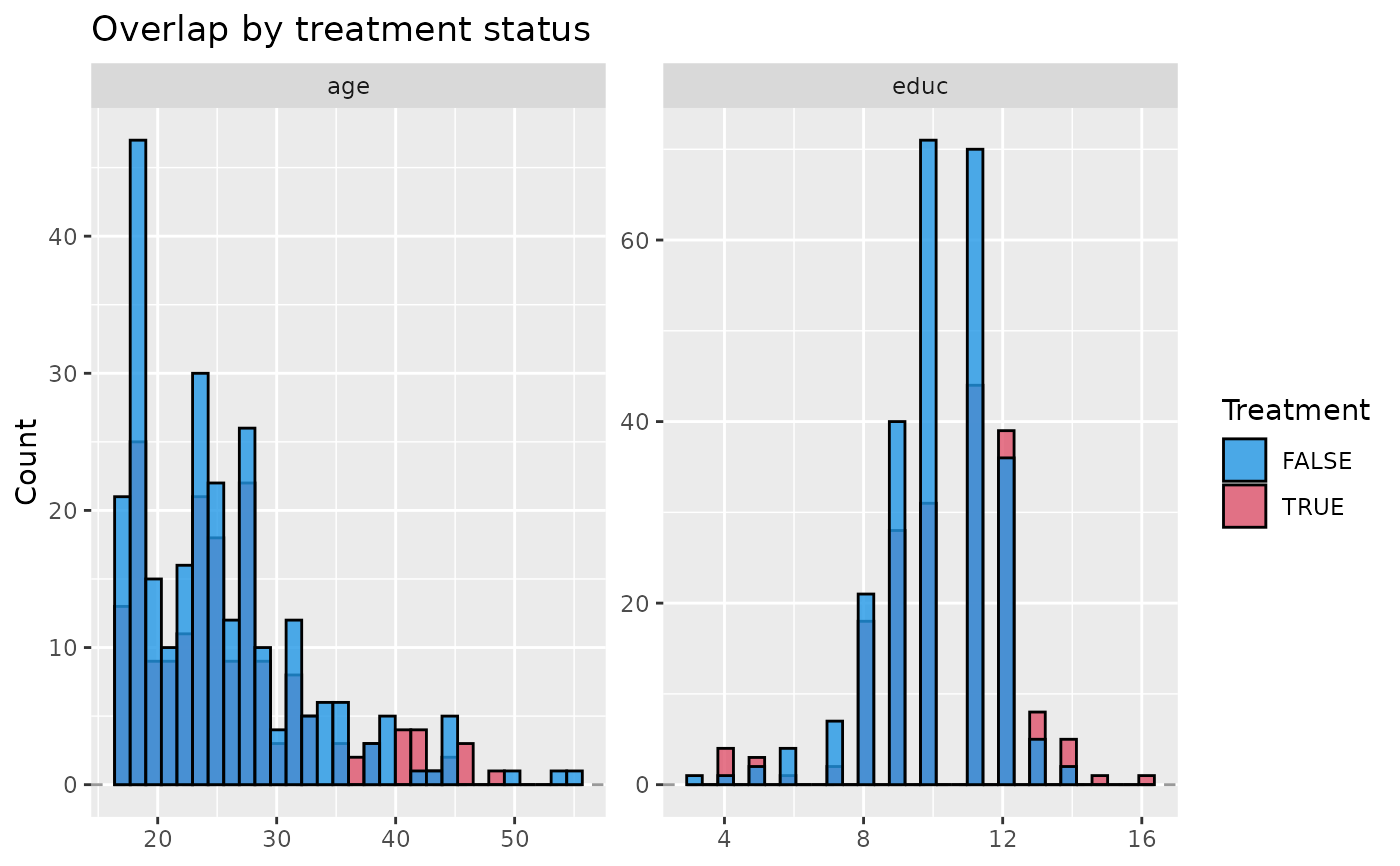Plot histograms showing the overlap between variables by treatment status.

## Usage

plot_overlap_vars(
.data,
treatment,
confounders,
plot_type = c("histogram", "density"),
min_x = NULL,
max_x = NULL
)

## Arguments

.data

dataframe

treatment

character. Name of the treatment column within .data

confounders

character list of column names denoting confounders within .data

plot_type

the plot type, one of c('histogram', 'density'). Defaults to 'histogram'

min_x

numeric value specifying the minimum value to be shown on the x axis

max_x

numeric value specifying the maximum value to be shown on the x axis

## Value

ggplot object

plot_overlap_pScores

## Author

George Perrett, Joseph Marlo

## Examples

data(lalonde)
plot_overlap_vars(
.data = lalonde,
treatment = 'treat',
confounders = c('age', 'educ'),
plot_type = 'Histogram'
)
#> stat_bin() using bins = 30. Pick better value with binwidth.
#> stat_bin() using bins = 30. Pick better value with binwidth.